## Ideal and real Success Sets

The similarity-function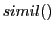presupposes a relationship between the actual working set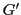as well as the ideal success set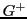. But the question is, how such a relationship should look like. We have the additional postulate that there must exist some ordering between the elements of the image set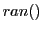of the evaluation function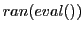as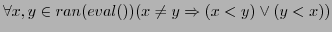, otherwise the fitness values can give no 'hint' for an optimization.

For an evaluation function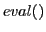I have to assume elements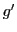of a working set. These elements must additionally be associated with some 'observable' = 'real' values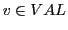in the world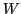which can be mapped onto a set of a 'success criterion' assumed as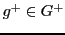. If we assume these success values as points in an n-dimensional space and the observable values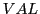as well, then we can compute some distance between some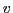and some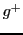with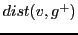. In this case it would be possible to enable a minimal ordering of the distance values if the set of success elements would not be completely randomly distributed. Thus there should exist some property of the setwhich can be 'computed' in a finite time.

In the case of a computable success setwe could then compute for every observable value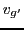a minimal distancesuch that the set of all distance sets ascould be ordered.

If the success set is not known in advance an observer can only count the number of the offspring. The number is then an 'indirect hint', a 'hidden index' for the success set. Thus the 'derived numbers'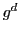fromwould be an empirical estimate for the ideal success set. The derived numbers should also obey an ordering. Thusis the set of all derived success values as an empirical estimate of.

'Not knowing the success setin advance' characterizes the case of the 'real' world; otherwise we are in an 'ideal' world where the success set is a purely theoretical entity in a 'simulated (= virtual)' world.

Gerd Doeben-Henisch 2013-01-14